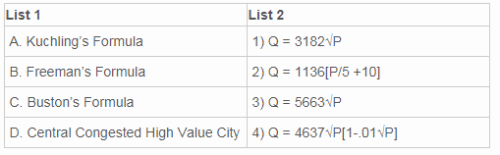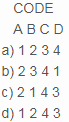# Test: Water Demand

## 15 Questions MCQ Test Environmental Engineering | Test: Water Demand

Description
Attempt Test: Water Demand | 15 questions in 25 minutes | Mock test for Chemical Engineering preparation | Free important questions MCQ to study Environmental Engineering for Chemical Engineering Exam | Download free PDF with solutions
QUESTION: 1

### The average quantity of water (in lpcd) required for domestic purposes according to IS code is ________

Solution:

Explanation: The average domestic water demand in India is 135 litres per capita per day. For developed countries, average domestic water demand is around 340 lpcd because they live a luxurious life.

QUESTION: 2

### The average consumption of water required in factories in lpcd is ___________

Solution:

Explanation: The average water consumption in factories is 30lpcd when no bathrooms are required and 45lpcd when bathrooms are required.

QUESTION: 3

### In which type of water demand, minimum average consumption of water takes place?

Solution:

Explanation: The minimum average water consumption takes place in fire demand. It accounts to 1lpcd which is very less but this water is required in very less duration.

QUESTION: 4

What is the minimum water pressure available at fire hydrants?

Solution:

Explanation: The minimum water presure available at fire hydrants is 100-150kN/m² i.e 10-15m of water head and it should be maintained for 4 to 5 hours.

QUESTION: 5

Match list 1(calculation of fire demand) with list 2 (formulae) and select the correct answer using the codes given below.where Q = quantity of water, P = population in thousandsSolution:

Explanation: Kuchling’s formula is used when the population of the city exceeds 50,000.
When the population of the city is less than 2 lakh, the above formula for central congested high value city is used.

QUESTION: 6

What is the fire demand of the city of 1lakh population by Buston’s formula?

Solution:

Explanation: By Buston’s formula , Q = 5663√P where P in thousands. Here, P =100, Q = 5663√100 => Q = 56630.

QUESTION: 7

Water lost in theft and waste contributes to how much % of total consumption?

Solution:

Explanation: Water loss in thefts and wastes contributes to 15% of total consumption, which includes the water loss due to leakage or stolen due to illegal connection.

QUESTION: 8

Which is the correct statement regarding per capita demand?

Solution:

Explanation: The per capita demand is the annual average amount of daily water required by one person. It is denoted by q in litres per day per person.

QUESTION: 9

What are the factors affecting per capita demand?

Solution:

Explanation: Increase in cost of water, quality of water and size of the city will result in more consumption of water. Poor habits of people in the utilization of water will also cause greater consumption.

QUESTION: 10

Which of the following statement is correct?

Solution:

Explanation: Rich class consumes more water, loss of water is more if the pressure in distribution system is more, water consumption is more in flush system and b option is the only correct statement.

QUESTION: 11

If the annual average hourly demand of the city is 10000m3, what is the maximum hourly consumption?

Solution:

Explanation: Maximum hourly demand = 2.7 * annual average hourly demand
=2.7 * 10000
=27000m3.

QUESTION: 12

If in a city, the maximum daily draft is 25MLD, fire draft is 35MLD and maximum hourly draft is 40MLD, what is the coincident draft?

Solution:

Explanation: Maximum daily draft +fire draft = 25 + 35 = 60MLD
Maximum hourly draft = 40MLD
Coincident draft is maximum of maximum draft draft + fire draft and maximum hourly draft =60MLD.

QUESTION: 13

What is the design period for the water treatment unit?

Solution:

Explanation: Water treatment unit is designed for 15 years and its design discharge is a maximum daily demand.

QUESTION: 14

What is the design discharge for intake structures?

Solution:

Explanation: Intake structures and the pipe mains that carry water from intake structures to treatment plant are designed for maximum daily demand.

QUESTION: 15

In which of the following units, design period is maximized?

Solution:

Explanation: Distribution system is designed for 30 years, the demand reservoir for 50 years, the water treatment unit for 15 years and the pipe mains for 30 years.
A B C D
a) 1 2 3 4
b) 2 3 4 1
c) 2 1 4 3
d) 1 2 4 3Use Code STAYHOME200 and get INR 200 additional OFF Use Coupon Code